# 1 Prior Knowledge Level A Level B Level

• Slides: 19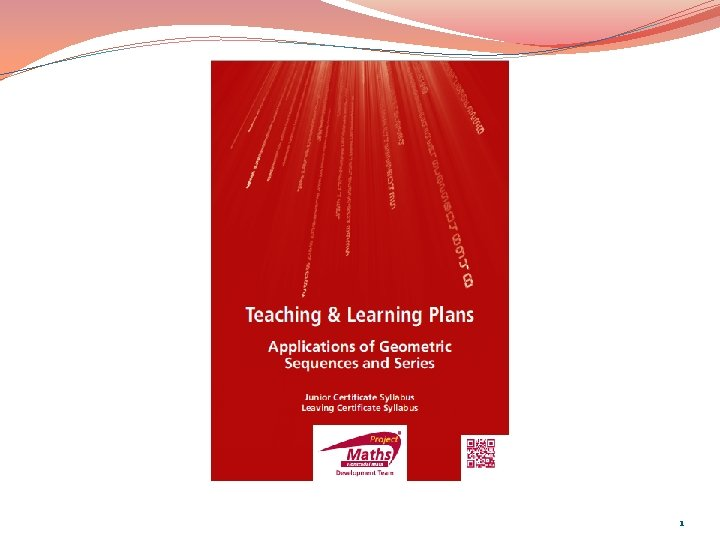1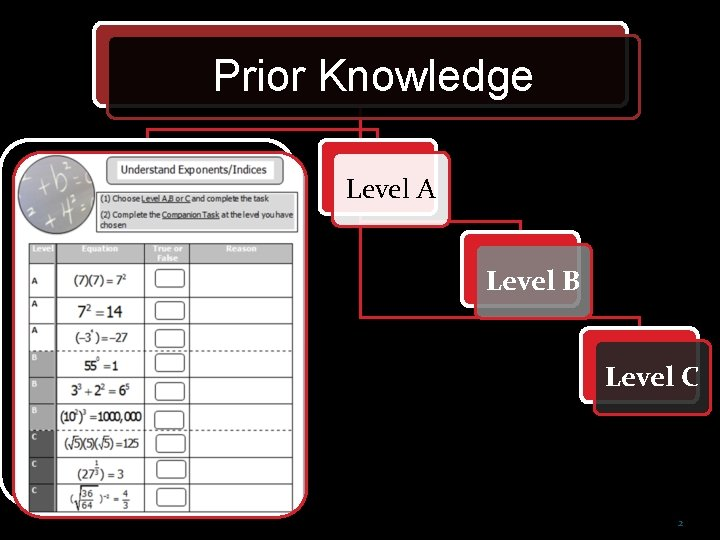Prior Knowledge Level A Level B Level C 2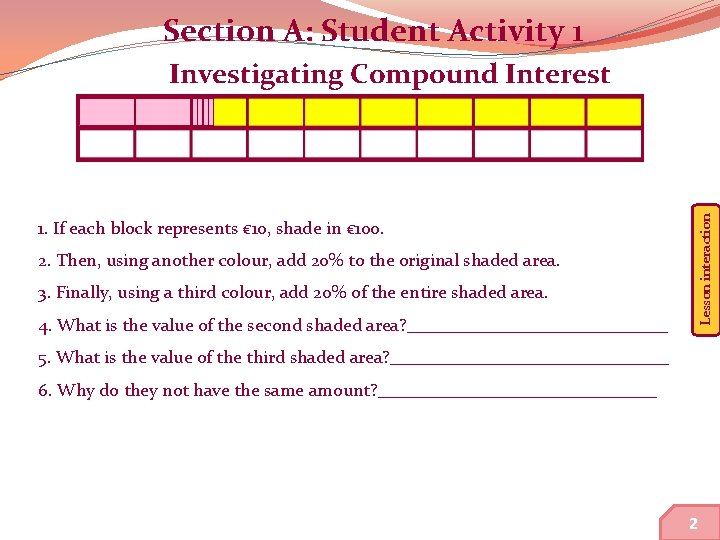Section A: Student Activity 1 Lesson interaction Investigating Compound Interest 1. If each block represents € 10, shade in € 100. 2. Then, using another colour, add 20% to the original shaded area. 3. Finally, using a third colour, add 20% of the entire shaded area. 4. What is the value of the second shaded area? _______________ 5. What is the value of the third shaded area? ________________ 6. Why do they not have the same amount? ________________ 5 2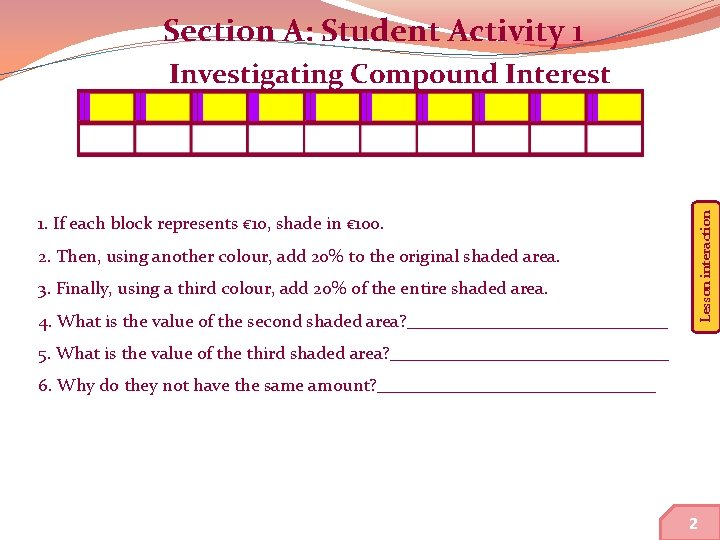Section A: Student Activity 1 Lesson interaction Investigating Compound Interest 1. If each block represents € 10, shade in € 100. 2. Then, using another colour, add 20% to the original shaded area. 3. Finally, using a third colour, add 20% of the entire shaded area. 4. What is the value of the second shaded area? _______________ 5. What is the value of the third shaded area? ________________ 6. Why do they not have the same amount? ________________ 6 2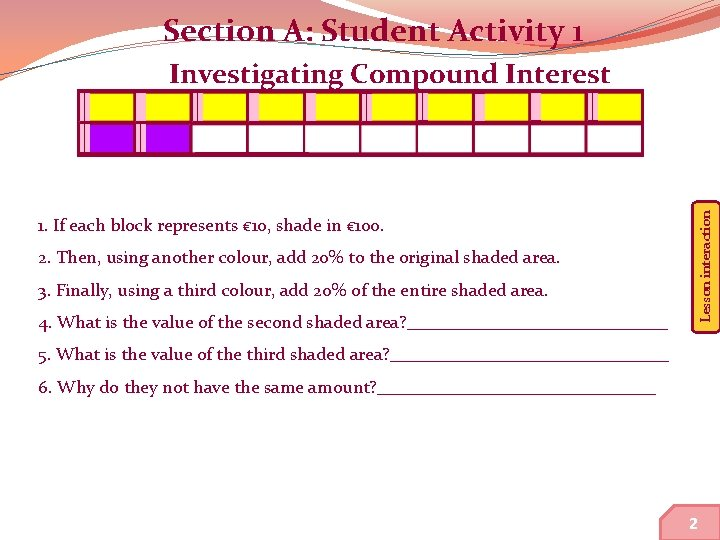Section A: Student Activity 1 Lesson interaction Investigating Compound Interest 1. If each block represents € 10, shade in € 100. 2. Then, using another colour, add 20% to the original shaded area. 3. Finally, using a third colour, add 20% of the entire shaded area. 4. What is the value of the second shaded area? _______________ 5. What is the value of the third shaded area? ________________ 6. Why do they not have the same amount? ________________ 7 2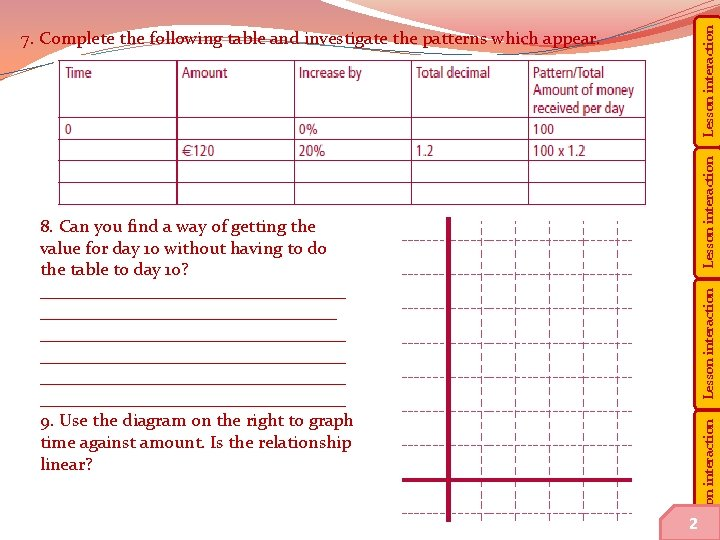Lesson interaction 7. Complete the following table and investigate the patterns which appear. 8 2 Lesson interaction 8. Can you find a way of getting the value for day 10 without having to do the table to day 10? __________________________________ __________________________________ 9. Use the diagram on the right to graph time against amount. Is the relationship linear?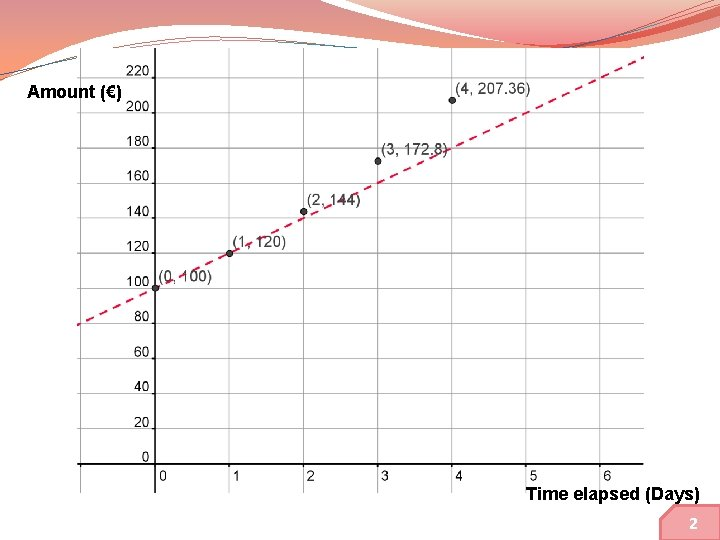Amount (€) Time elapsed (Days) 9 2Amount (€) Time Amount 0 100 1 120 2 144 3 172. 80 4 207. 36 Change 20 24 28. 80 34. 56 Change of Change 4 4. 80 5. 76 Time elapsed (Days) 2Amount (€) Ch Time Amount Multiplier Ch 0 100 1 120 2 144 3 172. 80 4 207. 36 1. 2 Time elapsed (Days) 2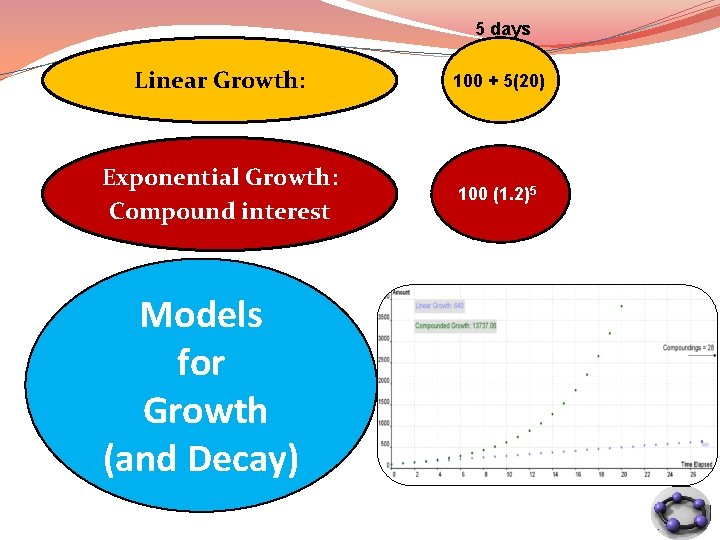5 days Linear Growth: 100 + 5(20) Exponential Growth: Compound interest 100 (1. 2)5 Models for Growth (and Decay) 12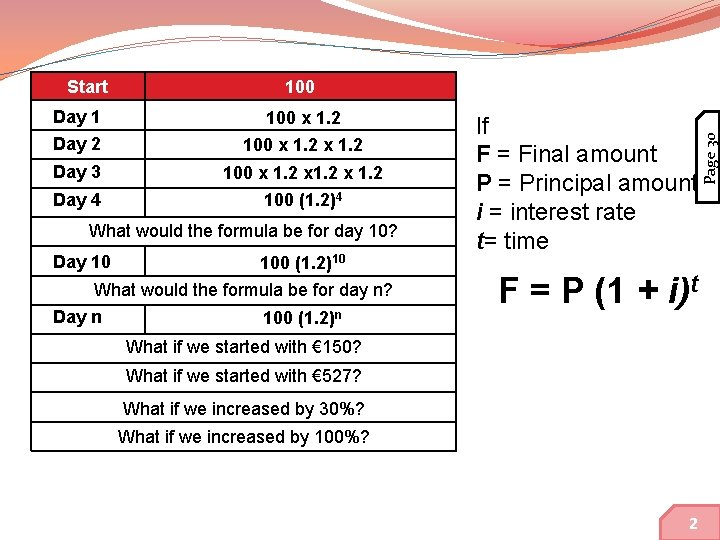100 Day 1 100 x 1. 2 Day 2 100 x 1. 2 Day 3 100 x 1. 2 Day 4 100 (1. 2)4 What would the formula be for day 10? Day 10 100 (1. 2)10 What would the formula be for day n? Day n 100 (1. 2)n If F = Final amount P = Principal amount i = interest rate t= time F = P (1 + i)t What if we started with € 150? What if we started with € 527? What if we increased by 30%? What if we increased by 100%? 13 2 Page 30 StartPage 30 Compound Interest Formula 14 2Section E Student Activity 5 Reducing Balance 15Lesson interaction Index Section A Section B Section C Section D Section E Appendix Section E: Student Activity 5 Reducing Balance David and Michael are going on the school tour this year. They are each taking out a loan of € 600, which they hope to pay off over the next year. Their bank is charging a monthly interest rate of 1. 5% on loans. David says that with his part time work at present he will be able to pay € 100 for the first 4 months but will only be able to pay off € 60 a month after that. Michael says that he can only afford to pay € 60 for the first 4 months and then € 100 after that. Michael reckons that they are both paying the same amount for the loan. Why? ____________________________________________________________ Note: This problem is posed based on the following criteria: (a) A loan is taken out (b) After 1 month interest is added on (c) The person then makes his/her monthly repayment. This process is then repeated until the loan is fully paid off. 16 2Lesson interaction Index Section A Section B Section C Section D Section E Appendix David Michael Time Monthly Total Interest Payments Time 0 600 9 100 0 1 509 Monthly Total Interest Payments 1 2 2 3 3 4 4 5 5 6 6 7 7 8 8 9 9 10 10 18 3Lesson interaction Index Section A Section B Section C Section D Section E Appendix 1. What do David and Michael have in common at the beginning of the loan period? _______________________ 2. Calculate the first 3 months transactions for each. (How much, in total, had they each paid back after 3 months? ) David ______ Michael _____ 3. What is the total interest paid by each? David _____ Michael _____ 4. Based on your answers to the first 3 questions, when would you recommend making the higher payments and why? _______________________________ 5. Is Michael’s assumption that they will eventually pay back the same amount valid? ________________________ 19 3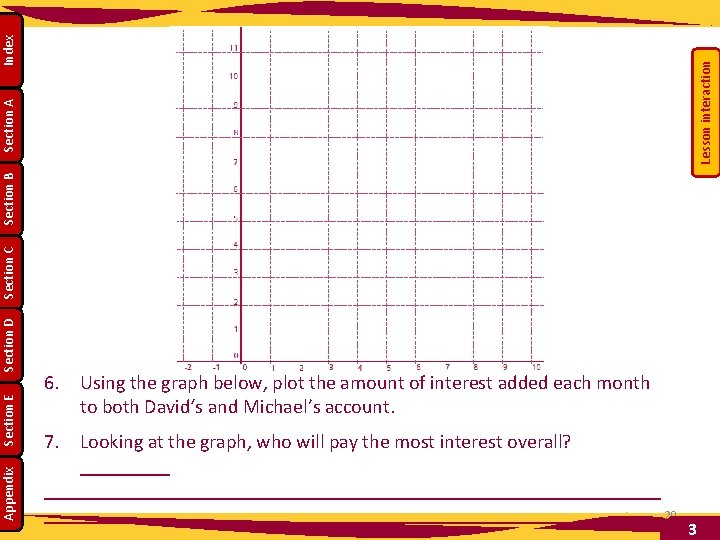Lesson interaction Index Section A Section B Section C Section D Section E Appendix 6. Using the graph below, plot the amount of interest added each month to both David‘s and Michael’s account. 7. Looking at the graph, who will pay the most interest overall? ____________________________________ 20 3Amount of interest paid (€) Michael David Time elapsed (months) 21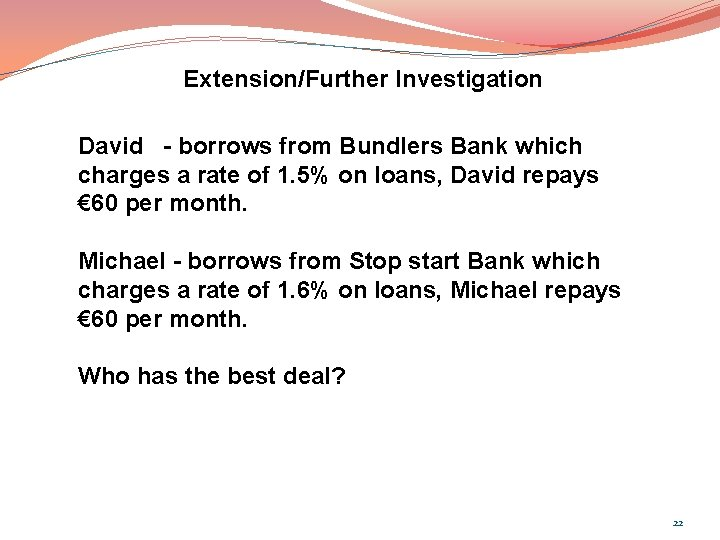Extension/Further Investigation David - borrows from Bundlers Bank which charges a rate of 1. 5% on loans, David repays € 60 per month. Michael - borrows from Stop start Bank which charges a rate of 1. 6% on loans, Michael repays € 60 per month. Who has the best deal? 22